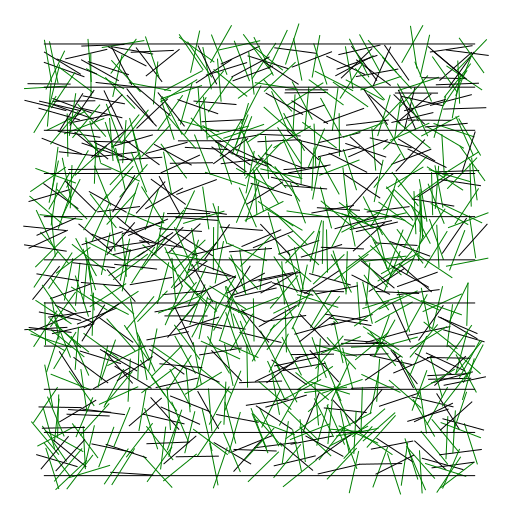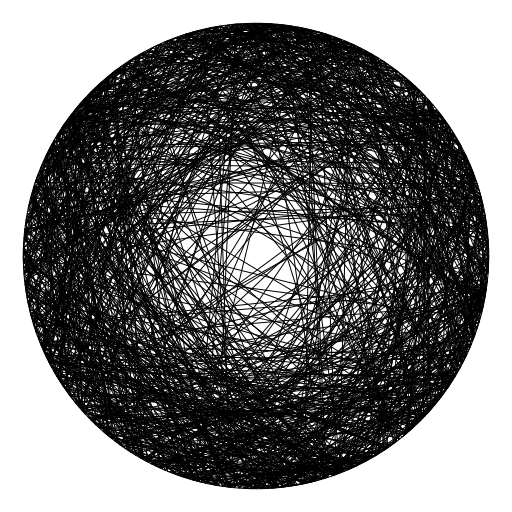# Buffon's Needle and Bertrand's Paradox¶

In []:
# If you don't have the package Graphics2D installed,
# Run the following command to get it
# Pkg.clone("git://github.com/sswatson/Graphics2D.jl.git")

In :
using Graphics2D

In :
using Gadfly

In :
# n is the size of the box in which we're tossing needles
n = 10

Out:
10

In :
# generates a random triple of real numbers representing a needle
# of the form (xposition,yposition,angle)
function needle(n)
return (n*rand(), n*rand(), π*rand())
end

function drawneedle(t)
return Line([t-0.5*cos(t) t-0.5*sin(t); t+0.5*cos(t) t+0.5*sin(t)];
color =  hitline(t) ? green*0.5 : black )
end

# returns true or flase depending on whether the needle is hitting a line
function hitline(t)
return min(t-floor(t),1-(t-floor(t))) < sin(t)/2
end

Out:
hitline (generic function with 1 method)

In :
numtrials = 1000
showgraphics([[drawneedle(needle(n)) for _=1:numtrials],[Line([0 k; n k]) for k=0:n]])

Out:In :
numtrials = 1_000_000
2/(sum([hitline(needle(n)) for _=1:numtrials])/numtrials)

Out:
3.1428652245105773

In :
π

Out:
π = 3.1415926535897...


In :
# Chose the chord's midpoint from area measure on the disk
function randomchord1()
x,y = 2*rand()-1, 2*rand()-1
if x^2 + y^2 < 1
θ = angle(x+im*y)
ψ = acos(hypot(x,y))
return [(cos(θ-ψ),sin(θ-ψ)),(cos(θ+ψ),sin(θ+ψ))]
else
return randomchord1()
end
end

# Choose the chord's midpoint's polar coordinates
# from area measure on the rectangle [0,1] x [0,2π]
function randomchord2()
r,θ = rand(), 2π*rand()
x,y = r*cos(θ), r*sin(θ)
if x^2 + y^2 < 1
θ = angle(x+im*y)
ψ = acos(hypot(x,y))
return [(cos(θ-ψ),sin(θ-ψ)),(cos(θ+ψ),sin(θ+ψ))]
else
return randomchord2()
end
end

# Choose the angles θ and φ of the endpoints of the chord
# from area measure on [0,2π] x [0,2π]
function randomchord3()
θ,φ = 2π*rand(), 2π*rand()
return [(cos(θ),sin(θ)),(cos(φ),sin(φ))]
end

Out:
randomchord3 (generic function with 1 method)

In :
showgraphics([[Line(randomchord1()) for _=1:1000],[Circle((0,0),1)]])

Out:In :
showgraphics([[Line(randomchord2()) for _=1:1000],[Circle((0,0),1)]])

Out: